# 1.First of all

Practice this to improve the technical capabilities of image preprocessing 100 knocks for image processing !! I will do it with Colaboratory so that it is easy to get started. We will work to complete the goal in two weeks. I will explain it carefully. Please ask a question! 001 --010 is the link on the right Knock 100 image processing !! (001 --010) Carefully and carefully 011 --020 is the link on the right 100 knocks on image processing !! (011 --020) Early game

# 2. Preparation

Introduced libraries etc. as follows.

#### `python`

``````
#Import library
import numpy as np
import matplotlib.pyplot as plt
import cv2

#Grayscale image
gray = cv2.cvtColor(img, cv2.COLOR_BGR2GRAY)
gray_noise = cv2.cvtColor(img_noise, cv2.COLOR_BGR2GRAY)
gray_dark = cv2.cvtColor(img_dark, cv2.COLOR_BGR2GRAY)
#For image storage
OUT_DIR = 'Output destination path/OUTPUT/'
``````

# 3. Explanation

## Q.21. Histogram normalization

Implement histogram normalization. It can be seen that the histogram has a bias. For example, if there are many pixels close to 0, the image will be dark overall, and if there are many pixels close to 255, the image will be bright. Locally biased histograms are expressed as having a narrow dynamic range. Therefore, in order to make the image easier for the human eye to see, it is necessary to perform processing such as normalizing or flattening the histogram. This histogram normalization is called gray-scale transformation, and when converting an image with a pixel value of [c, d] to the range of [a, b], it can be realized by the following equation. This time, imori_dark.jpg is converted to the range of [0, 255] respectively.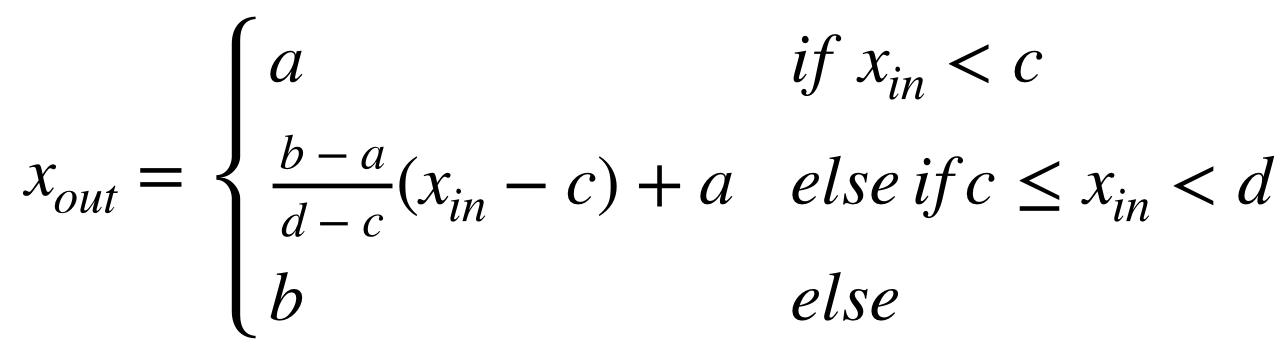#### `A21`

``````
def hist_normalization(img, a=0, b=255):
"""
Histogram normalization
params
----------------------------
param1: numpy.ndarray format image
param2:Minimum histogram range
param3:Maximum histogram range

returns
----------------------------
numpy.ndarray format image
"""
#histogram(rgb)Maximum / minimum value of
c = img.min()     # 60
d = img.max()    # 141

#copy
out = img.copy()

#Normalization
out = (b - a) / (d - c) * (out - c) + a
out[out < a] = a
out[out > b] = b
out = out.astype(np.uint8)
return out

#Get image height, width and color
H, W, C = img_dark.shape

#Histogram normalization
out = hist_normalization(img_dark)

#Show histogram
plt.hist(out.ravel(), bins=255, rwidth=0.8, range=(0, 255))
plt.savefig("img21.png ")
plt.show()
``````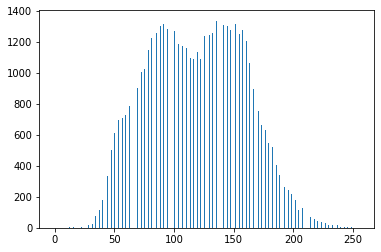![img21_.png](https://qiita-image-store.s3.ap-northeast-1.amazonaws.com/0/621150/52139d68-fc5d-0658-b887-7badda5ebcc9.png) The image is also pretty clear.

Reference: Histogram # 2: Histogram flattening

## Q.22. Histogram operation

Operate so that the mean value of the histogram is m0 = 128 and the standard deviation is s0 = 52. This is an operation that changes the histogram flat rather than changing the dynamic range of the histogram. To change the histogram of the mean value m and standard deviation s to the mean value m0 and standard deviation s0, convert by the following equation.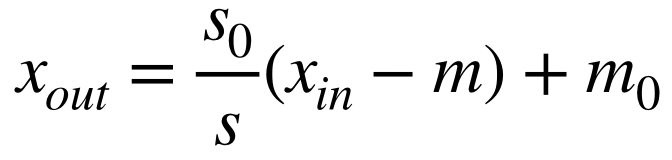#### `A22`

``````
def hist_mani(img, m0=128, s0=52):
"""
The mean value of the histogram is m0=128, standard deviation s0=Operate to be 52

params
--------------------------------------
param1: numpy.ndarray format image
param2:Average value
param3:standard deviation

returns
--------------------------------------
numpy.ndarray format image
"""
#Average value
m = np.mean(img)
#standard deviation
s = np.std(img)

#Copy of image
out = img.copy()

#Calculate according to the formula
out = s0 / s * (out - m) + m0
out[out < 0] = 0
out[out > 255] = 255
out = out.astype(np.uint8)

return out

#Manipulate the histogram
out = hist_mani(img_dark)

#Save the result
cv2.imwrite(OUT_DIR + 'ans22_1.jpg', out)
#Display image
cv2_imshow(out)
cv2.waitKey(0)
cv2.destroyAllWindows()

#Show histogram
plt.hist(out.ravel(), bins=255, rwidth=0.8, range=(0, 255))
plt.savefig("img22_2.png ")
plt.show()
``````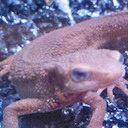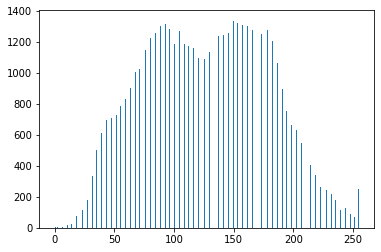Reference: Histogram # 2: Histogram flattening

## Q.23. Histogram flattening

Implement histogram flattening. Histogram flattening is an operation of changing the histogram flat, and is an operation of balancing the histogram values without requiring the above-mentioned average value and standard deviation. This is defined by the following equation. However, S ... the total number of pixel values, Zmax ... the maximum value of pixel values, h (z) ... the frequency of density z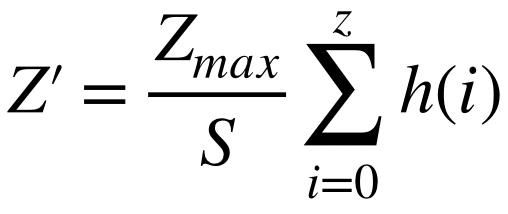#### `A23`

``````
def hist_equal(img, z_max=255):
"""
Histogram flattening

params
--------------------------------------
param1: numpy.ndarray format image
param2:Maximum pixel value

returns
--------------------------------------
numpy.ndarray format image
"""

#Get image height, width and color
H, W, C = img.shape
#Total number of pixel values(Image height x image width x number of colors)
S = H * W * C * 1.     # 49152.0
#Copy of image
out = img.copy()

#Concentration frequency
sum_h = 0.

#Image density 0~Each frequency up to 255
for i in range(256):
#Where the concentrations match
ind = np.where(img==i)
#Concentration frequency
sum_h += len(img[ind])
#Balance histogram values(Refer to the formula)
z_prime = z_max / S * sum_h
out[ind] = z_prime

out = out.astype(np.uint8)

return out

#Manipulate the histogram
out = hist_equal(img)

#Save the result
cv2.imwrite(OUT_DIR + 'ans23_1.jpg', out)
#Display image
cv2_imshow(out)
cv2.waitKey(0)
cv2.destroyAllWindows()

#Show histogram
plt.hist(out.ravel(), bins=255, rwidth=0.8, range=(0, 255))
plt.savefig("img23_2.png ")
plt.show()
``````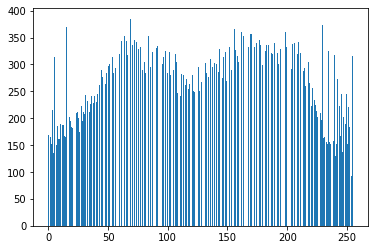Reference: Histogram # 2: Histogram flattening

## Q.24. Gamma correction

Perform gamma correction (c = 1, g = 2.2) on imori_gamma.jpg. Gamma correction is correction when pixel values are non-linearly converted via a medium such as a camera. If the image is displayed as it is on a display or the like, the screen becomes dark. Therefore, the purpose of gamma correction is to display an image excluding the characteristics of the display by increasing the RGB value in advance. Non-linear transformation is said to occur by the following equation. However, x is normalized to [0,1]. c ... constant, g ... gamma characteristic (usually 2.2)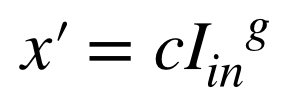Therefore, gamma correction is performed by the following equation.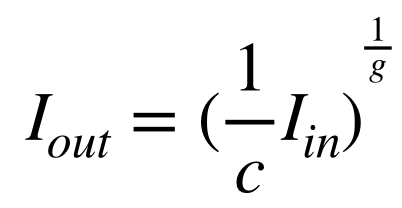#### `A24`

``````
def gamma_correction(img, c=1, g=2.2):
"""
Gamma correction: How to adjust the brightness of an image

params
--------------------------------------
param1: numpy.ndarray format image
param2:constant
param3:Gamma characteristics

returns
--------------------------------------
numpy.ndarray format image
"""

#Copy of image
out = img.copy().astype(np.float)
#Divide by 255(Convert to Iin)
out /= 255.
#Gamma correction formula
out = (1/c * out) ** (1/g)

#Multiply 255
out *= 255
out = out.astype(np.uint8)

return out

#Gamma correction
out = gamma_correction(img_gamma)

#Save the result
cv2.imwrite(OUT_DIR + 'ans24.jpg', out)
#Display image
cv2_imshow(out)
cv2.waitKey(0)
cv2.destroyAllWindows()
``````Reference: Try Python!

## Q.25. Nearest neighbor interpolation

Enlarge the image 1.5 times by nearest neighbor interpolation. Nearest Neighbor is a method that uses the pixels in the nearest neighbor as they are when enlarging the image. It is simple and the processing speed is fast, but the image quality deteriorates significantly. Interpolated by the following equation. I'... image after enlargement, I ... image before enlargement, a ... magnification, [] ... rounding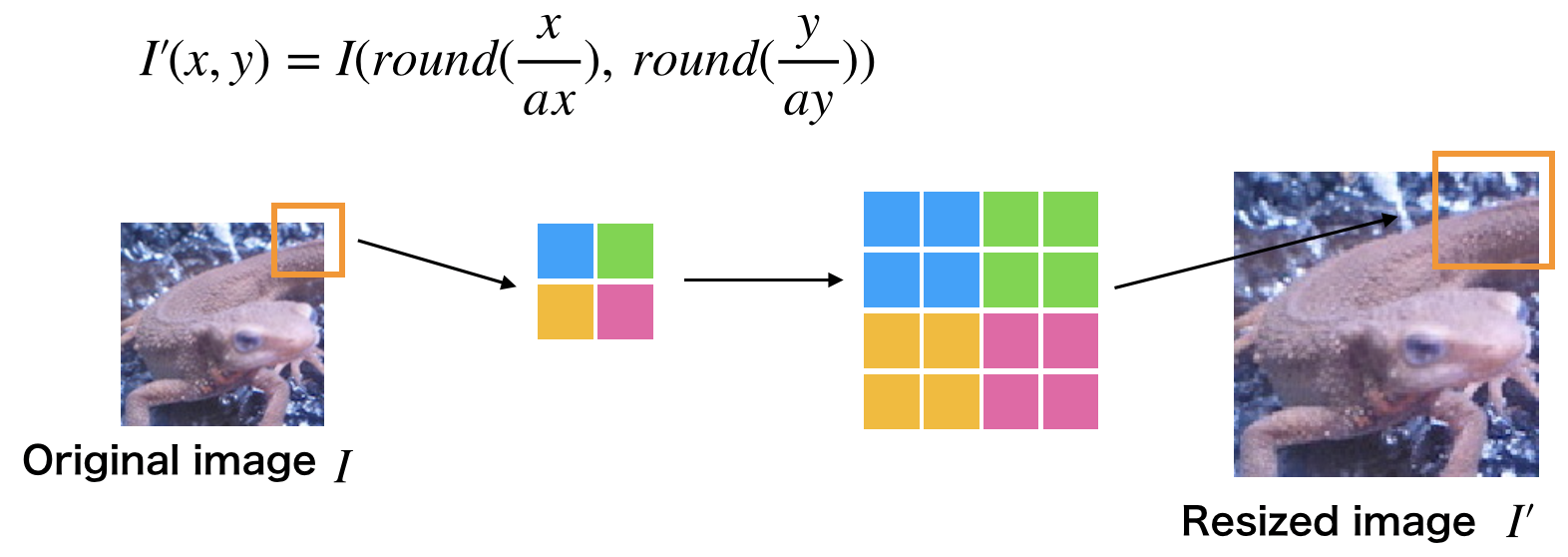#### `A25`

``````
"""
Nearest neighbor interpolation
cv2.resize(src, dsize[, interpolation])
src input image
Image size after changing dsize
interpolation Interpolation method(Nearest neighbor interpolation is cv2.INTER_NEAREST)
"""
#Nearest neighbor interpolation
#Image size after change: img.shape>>>(Height, width, color)
out = cv2.resize(
img, (int(img.shape*1.5), int(img.shape*1.5)), interpolation=cv2.INTER_NEAREST)

#Save the result
cv2.imwrite(OUT_DIR + 'ans25.jpg', out)
#Display image
cv2_imshow(out)
cv2.waitKey(0)
cv2.destroyAllWindows()
``````Reference: [[Python / OpenCV] Enlargement / reduction of image (nearest neighbor interpolation method, bilinear interpolation method, bicubic interpolation method)](https://algorithm.joho.info/programming/python/opencv-resize-nearest- interpolation-py /)

## Q.26. Bi-linear interpolation

Enlarge the image 1.5 times with Bi-linear interpolation. Bi-linear interpolation is a method of complementing the surrounding four pixels by weighting them according to the distance. The larger the amount of calculation, the longer the processing time, but the deterioration of image quality can be suppressed.

#### `A26`

``````
"""
Bilinear interpolation method (Bi-linear interpolation) is an interpolation method that uses the four surrounding pixels.
cv2.resize(src, dsize[, interpolation])
src input image
Image size after changing dsize
interpolation Interpolation method(Cv2 for bilinear interpolation.INTER_LINEAR)
"""
#Bilinear interpolation method
#Image size after change: img.shape>>>(Height, width, color)
out = cv2.resize(
img, (int(img.shape*1.5), int(img.shape*1.5)), interpolation=cv2.INTER_LINEAR)

#Save the result
cv2.imwrite(OUT_DIR + 'ans26.jpg', out)
#Display image
cv2_imshow(out)
cv2.waitKey(0)
cv2.destroyAllWindows()
``````Reference: [[Python / OpenCV] Enlargement / reduction of image (nearest neighbor interpolation method, bilinear interpolation method, bicubic interpolation method)](https://algorithm.joho.info/programming/python/opencv-resize-nearest- interpolation-py /)

## Q.27. Bi-cubic interpolation

Enlarge the image 1.5 times with Bi-cubic interpolation. Bi-cubic interpolation is an extension of Bi-linear interpolation, and interpolation is performed from the surrounding 16 pixels.

#### `A27`

``````
"""
The bicubic interpolation method uses the pixel values of 16 surrounding pixels.
cv2.resize(src, dsize[, interpolation])
src input image
Image size after changing dsize
interpolation Interpolation method(Cv2 for bicubic interpolation.INTER_CUBIC)
"""
#Bicubic interpolation
#Image size after change: img.shape>>>(Height, width, color)
out = cv2.resize(
img, (int(img.shape*1.5), int(img.shape*1.5)), interpolation=cv2.INTER_CUBIC)

#Save the result
cv2.imwrite(OUT_DIR + 'ans27.jpg', out)
#Display image
cv2_imshow(out)
cv2.waitKey(0)
cv2.destroyAllWindows()
``````Reference: [[Python / OpenCV] Enlargement / reduction of image (nearest neighbor interpolation method, bilinear interpolation method, bicubic interpolation method)](https://algorithm.joho.info/programming/python/opencv-resize-nearest- interpolation-py /)

## Q.28. Affine transformation (translation)

Use the affine transformation to translate the image by +30 in the x direction and -30 in the y direction. Affine transformation is an operation that transforms an image using a 3x3 matrix.

#### `A28`

``````
"""
Affine transformation
cv2.warpAffine(src, M, dsize[, dst[, flags[, borderMode[, borderValue]]]])
Original image (NumPy array ndarray) as the first argument,
2 x 3 transformation matrix (NumPy array ndarray) as the second argument,
Specify the size (tuple) of the output image in the third argument.
"""
#Get image height, width and color
H, W, C = img.shape
#Translation[[1,0,Amount of movement in the lateral direction],[0,1,Amount of movement in the vertical direction]]2x3 matrix
M = np.float64([[1, 0, 30], [0,1,-30]])
#Affine transformation
out = cv2.warpAffine(img, M, (W, H))

#Save the result
cv2.imwrite(OUT_DIR + 'ans28.jpg', out)
#Display image
cv2_imshow(out)
cv2.waitKey(0)
cv2.destroyAllWindows()
``````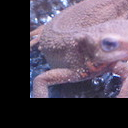## Q.29. Affine transformation (scaling)

Use the affine transformation to (1) resize 1.3 times in the x direction and 0.8 times in the y direction. Also, in addition to the conditions (2) and (1), realize parallel movement by +30 in the x direction and -30 in the y direction at the same time.

#### `A29`

``````

def affine_expand(img, ratio_x, ratio_y):
"""
Enlarge with affine transformation

params
-------------------------------
param1: numpy.ndarray format image
param2:Ratio in x direction
param3:Ratio in the y direction

returns
-------------------------------
numpy.ndarray format image
"""
#Image height and width
H, W = img.shape[:2]
#xy coordinates np.float32 type
src = np.array([[0.0, 0.0],[0.0, 1.0],[1.0, 0.0]], np.float32)
# x,y Multiply each ratio
dest = src.copy()
dest[:,0] *= ratio_x
dest[:,1] *= ratio_y
"""
Generate transformation matrix of affine transformation: cv2.getAffineTransform(src, dest)
src:Coordinates of 3 points before conversion
dest:Specify the coordinates of the three points after conversion with the NumPy array ndarray
"""
affine = cv2.getAffineTransform(src, dest)
"""
Affine transformation
cv2.warpAffine(src, M, dsize[, dst[, flags[, borderMode[, borderValue]]]])
Original image (NumPy array ndarray) as the first argument,
2 x 3 transformation matrix (NumPy array ndarray) as the second argument,
Specify the size (tuple) of the output image in the third argument.
INTER_LANCZOS4 – Lanczos interpolation using the neighborhood area of 8 × 8
"""
return cv2.warpAffine(img, affine, (int(W*ratio_x), int(H*ratio_y)), cv2.INTER_LANCZOS4) #Interpolation method can also be specified

#Enlarge with affine transformation
out = affine_expand(img, 1.3, 0.8)
#Translation[[1,0,Amount of movement in the lateral direction],[0,1,Amount of movement in the vertical direction]]2x3 matrix
H, W = out.shape[:2]
M = np.float64([[1, 0, 30], [0,1,-30]])
out2 = cv2.warpAffine(out, M, (W, H))

#Save the result
cv2.imwrite(OUT_DIR + 'ans29_1.jpg', out)
cv2.imwrite(OUT_DIR + 'ans29_2.jpg', out2)

#Display image
cv2_imshow(out)
cv2_imshow(out2)
cv2.waitKey(0)
cv2.destroyAllWindows()
``````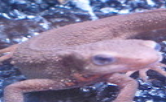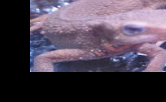## Q.30. Affine conversion (rotation)

(1) Rotate 30 degrees counterclockwise using affine transformation. (2) Use the affine transformation to fix the center coordinates of the image rotated 30 degrees counterclockwise, and create the image so that there are as few black areas as possible. (However, if you perform a simple affine transformation, the image will be cut off, so you need to be creative.)

#### `A30`

``````
def affin_rotate(img, x, y, theta, scale):
"""
Rotate with affine transformation

params
-------------------------------
param1: numpy.ndarray format image
param2:X coordinate of the axis of rotation
param3:Y coordinate of the axis of rotation
param4:Angle of rotation
param5:Rotation angle / magnification

returns
-------------------------------
numpy.ndarray format image
"""

"""
Affine transformation representing two-dimensional rotation
cv2.getRotationMatrix2D(center, angle, scale)
center:Coordinates that are the origin of rotation
angle:Angle of rotation (degrees, not radians)
scale:Enlargement / reduction magnification.
"""
#Calculation of rotation transformation matrix
R = cv2.getRotationMatrix2D((x, y), theta, scale)
"""
Affine transformation
cv2.warpAffine(src, M, dsize[, dst[, flags[, borderMode[, borderValue]]]])
Original image (NumPy array ndarray) as the first argument,
2 x 3 transformation matrix (NumPy array ndarray) as the second argument,
Specify the size (tuple) of the output image in the third argument.
cv2.INTER_CUBIC:Bicubic
"""
#Affine transformation
dst = cv2.warpAffine(img, R, gray.shape,
flags=cv2.INTER_CUBIC)

return dst

#Image center coordinates
oy, ox = int(img.shape/2), int(img.shape/2)

#Rotate 30 degrees counterclockwise
out1 = affin_rotate(img, 0, 0, 30, 1)
#Fixed center coordinates with an image rotated 30 degrees counterclockwise
out2 = affin_rotate(img, ox, oy, 30, 1)

#Save the result
cv2.imwrite(OUT_DIR + 'ans30_1.jpg', out1)
cv2.imwrite(OUT_DIR + 'ans31_2.jpg', out2)

#Display image
cv2_imshow(out1)
cv2_imshow(out2)
cv2.waitKey(0)
cv2.destroyAllWindows()
``````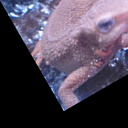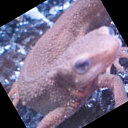# Impressions

It feels like the level is gradually rising. Try to implement in OpenCV as much as possible.